# OMPn: Orbital-Optimized Møller–Plesset Perturbation Theory¶

Code author: Ugur Bozkaya

Section author: Ugur Bozkaya

Module: Keywords, PSI Variables, OMP2

Module: Keywords, PSI Variables, OMP3

## Introduction¶

Orbital-optimized methods have several advantages over non-optimized counterparts. Once the orbitals are optimized, the wave function will obey the Hellmann-Feynman theorem for orbital rotation parameters. Therefore, there is no need for orbital response terms in the evaluation of analytic gradients. In other words, it is unnecessary to solve the first order coupled-perturbed CC and many-body perturbation theory (MBPT) equations. Further, computation of one-electron properties is easier because there are no response contributions to the particle density matrices (PDMs). Moreover, active space approximations can be readily incorporated into the CC methods [Krylov:2000:vod]. Additionally, orbital-optimized coupled-cluster avoids spurious second-order poles in its response function, and its transition dipole moments are gauge invarianti [Pedersen:1999:od].

Another advantage is that the orbital-optimized methods does not suffer from the artifactual symmetry-breaking instabilities [Sherrill:1998:od], [Bozkaya:2011:omp2], and [Bozkaya:2011:omp3]. Further, Kurlancheek and Head-Gordon [Kurlancek:2009] demonstrated that first order properties such as forces or dipole moments are discontinuous along nuclear coordinates when such a symmetry breaking occurs. They also observed that although the energy appears well behaved, the MP2 method can have natural occupation numbers greater than 2 or less than 0, hence may violate the N-representability condition. They further discussed that the orbital response equations generally have a singularity problem at the unrestriction point where spin-restricted orbitals become unstable to unrestriction. This singularity yields to extremely large or small eigenvalues of the one-particle density matrix (OPDM). These abnormal eigenvalues may lead to unphysical molecular properties such as vibrational frequencies. However, orbital optimized MP2 (hence Orbital optimized MP3) will solve this N-representability problem by disregarding orbital response contribution of one-partical density matrix.

Although the performance of coupled-cluster singles and doubles (CCSD) and orbital-optimized CCD (OD) is similar, the situation is different in the case of triples corrections, especially at stretched geometries [Bozkaya:2012:odtl]. Bozkaya and Schaefer demonstrated that orbital-optimized coupled cluster based triple corrections, especially those of asymmetrics, provide significantly better potential energy curves than CCSD based triples corrections.

## Theory¶

What follows is a very basic description of orbital-optimized Møller–Plesset perturbation theory as implemented in PSI4. We will follow our previous presentations ([Bozkaya:2011:omp2], [Bozkaya:2011:omp3], and [Bozkaya:2012:odtl])

The orbital variations may be expressed by means of an exponential unitary operator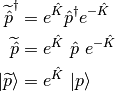where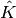is the orbital rotation operator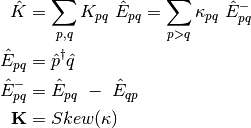The effect of the orbital rotations on the MO coefficients can be written as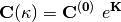where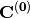is the initial MO coefficient matrix and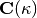is the new MO coefficient matrix as a function of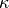. Now, let us define a variational energy functional (Lagrangian) as a function of• OMP2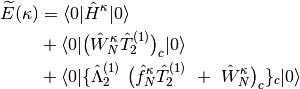• OMP3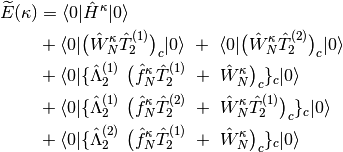where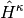,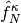, and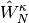defined as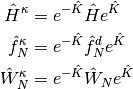and first and second derivatives of the energy with respect to theparameter at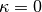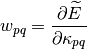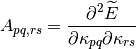Then the energy can be expanded up to second-order as follows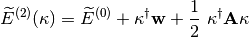where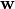is the MO gradient vector,is the MO rotation vector, and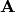is the MO Hessian matrix. Therefore, minimizing the energy with respect toyields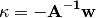This final equation corresponds to the usual Newton-Raphson step.

Publications resulting from the use of the OMP2 code should cite the following publication(s):

Publications resulting from the use of the OMP3 code should cite the following publications:

## Methods¶

The orbital-optimized MP2 methods currently supported in PSI4 are outlined in Table OMP2 Methods.

Name Calls Method Energy Gradient Reference
omp2 Orbital-Optimized MP2 Y Y RHF/UHF/RKS/UKS
scs-omp2 Spin-Component Scaled Orbital-Optimized MP2 Y N RHF/UHF/RKS/UKS
sos-omp2 Spin-Opposite Scaled Orbital-Optimized MP2 Y N RHF/UHF/RKS/UKS
scsn-omp2 A special version of SCS-OMP2 for nucleobase interactions Y N RHF/UHF/RKS/UKS
scs-mi-omp2 A special version of SCS-OMP2 (from S22 database) Y N RHF/UHF/RKS/UKS
scs-omp2-vdw A special version of SCS-OMP2 (from ethene dimers) Y N RHF/UHF/RKS/UKS
sos-pi-omp2 A special version of SOS-OMP2 for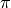-systems Y N RHF/UHF/RKS/UKS

The orbital-optimized MP3 methods currently supported in PSI4 are outlined in Table OMP3 Methods.

Name Calls Method Energy Gradient Reference
omp3 Orbital-Optimized MP3 Y N RHF/UHF/RKS/UKS
scs-omp3 Spin-Component Scaled Orbital-Optimized MP3 Y N RHF/UHF/RKS/UKS
sos-omp3 Spin-Opposite Scaled Orbital-Optimized MP3 Y N RHF/UHF/RKS/UKS
scsn-omp3 A special version of SCS-OMP3 for nucleobase interactions Y N RHF/UHF/RKS/UKS
scs-mi-omp3 A special version of SCS-OMP3 (from S22 database) Y N RHF/UHF/RKS/UKS
scs-omp3-vdw A special version of SCS-OMP3 (from ethene dimers) Y N RHF/UHF/RKS/UKS
sos-pi-omp3 A special version of SOS-OMP3 for-systems Y N RHF/UHF/RKS/UKS

## Basic Keywords¶

### E_CONVERGENCE¶

Convergence criterion for energy.

### R_CONVERGENCE¶

Convergence criterion for amplitudes (residuals).

Convergence criterion for RMS orbital gradient.

Convergence criterion for maximum orbital gradient

### MO_MAXITER¶

Maximum number of iterations to determine the orbitals

• Type: integer
• Default: 50

### OPT_METHOD¶

The optimization algorithm

• Type: string
• Possible Values: MSD, DIIS
• Default: MSD

### DIIS_MAX_VECS¶

Number of vectors used in DIIS

• Type: integer
• Default: 4

### LINEQ_SOLVER¶

The solver will be used for simultaneous linear equations.

• Type: string
• Possible Values: CDGESV, FLIN, POPLE
• Default: CDGESV

### ORTH_TYPE¶

The algorithm for orthogonalization of MOs

• Type: string
• Possible Values: GS, MGS
• Default: MGS

### MP2_OS_SCALE¶

MP2 opposite-spin scaling value

• Type: double
• Default: 6.0/5.0

### MP2_SS_SCALE¶

MP2 same-spin scaling value

• Type: double
• Default: 1.0/3.0

### SOS_SCALE¶

Spin-opposite scaling (SOS) value for SCF orbitals

• Type: double
• Default: 1.3

### SOS_SCALE2¶

Spin-opposite scaling (SOS) value for optimized-MP2 orbitals

• Type: double
• Default: 1.2

### NAT_ORBS¶

Do compute natural orbitals?

### OMP2_ORBS_PRINT¶

Do print OMP2 orbital energies?

### OMP3_ORBS_PRINT¶

Do print OMP3 orbital energies?

### TPDM_ABCD_TYPE¶

How to take care of the TPDM VVVV-block. The COMPUTE option means it will be computed via an IC/OOC algoritm. The DIRECT option (default) means it will not be computed and stored, instead its contribution will be directly added to Generalized-Fock Matrix.

• Type: string
• Possible Values: DIRECT, COMPUTE
• Default: DIRECT